﻿ 融合多幅海图的航线自动生成改进方法
«上一篇文章快速检索 高级检索

 哈尔滨工程大学学报2019, Vol. 40Issue (6): 1090-1097  DOI: 10.11990/jheu.2018030650

### 引用本文ZHANG Lihua, DAI Zeyuan, JIA Shuaidong. Improved method for route generation based on chart fusion[J]. Journal of Harbin Engineering University, 2019, 40(6), 1090-1097. DOI: 10.11990/jheu.201803065.### 文章历史

1. 海军大连舰艇学院 军事海洋与测绘系, 辽宁 大连 116018;
2. 海军大连舰艇学院 海洋测绘工程军队重点实验室, 辽宁 大连 116018

Improved method for route generation based on chart fusion
ZHANG Lihua 1,2, DAI Zeyuan 1,2, JIA Shuaidong 1,2
1. Department of Military Oceanography and Hydrography & Cartography, Dalian Naval Academy, Dalian 116018, China;
2. Key Laboratory of Hydrographic Surveying and Mapping of PLA, Dalian Naval Academy, Dalian 116018, China
Abstract: This work proposes an improved method for the automatic generation of routes in high-obstacle areas given the limitations of current route design methods that can only address a single chart. The proposed method is based on the fusion of multiple charts. Obstacle data are extracted from different charts for fusion and incremental update by quantitatively analyzing chart accuracy and timeliness differences. The spatial database for obstacle areas is established to construct the chart incremental updating mechanism. The route window and improved R-tree index are used to improve route generation efficiency by considering the impact of the data volume of multiple charts on the efficiency of route automatic generation and by applying the route binary tree algorithm. Experimental results show that the proposed method can automatically generate routes across charts with optimal safety and distance. The generated routes have been shortened by 9.8% and 21.8%, and the time consumption for route generation has reduced by 85.8% and 95.02%.
Keywords: chart    obstacle areas    route binary tree    shortest route    spatial database    data fusion    incremental update    improved R-tree

1 二叉树最短距离航线自动生成改进方法 1.1 现有方法的理论基础 1.1.1 现有方法的基本思想

1.1.2 单幅海图的碍航区表达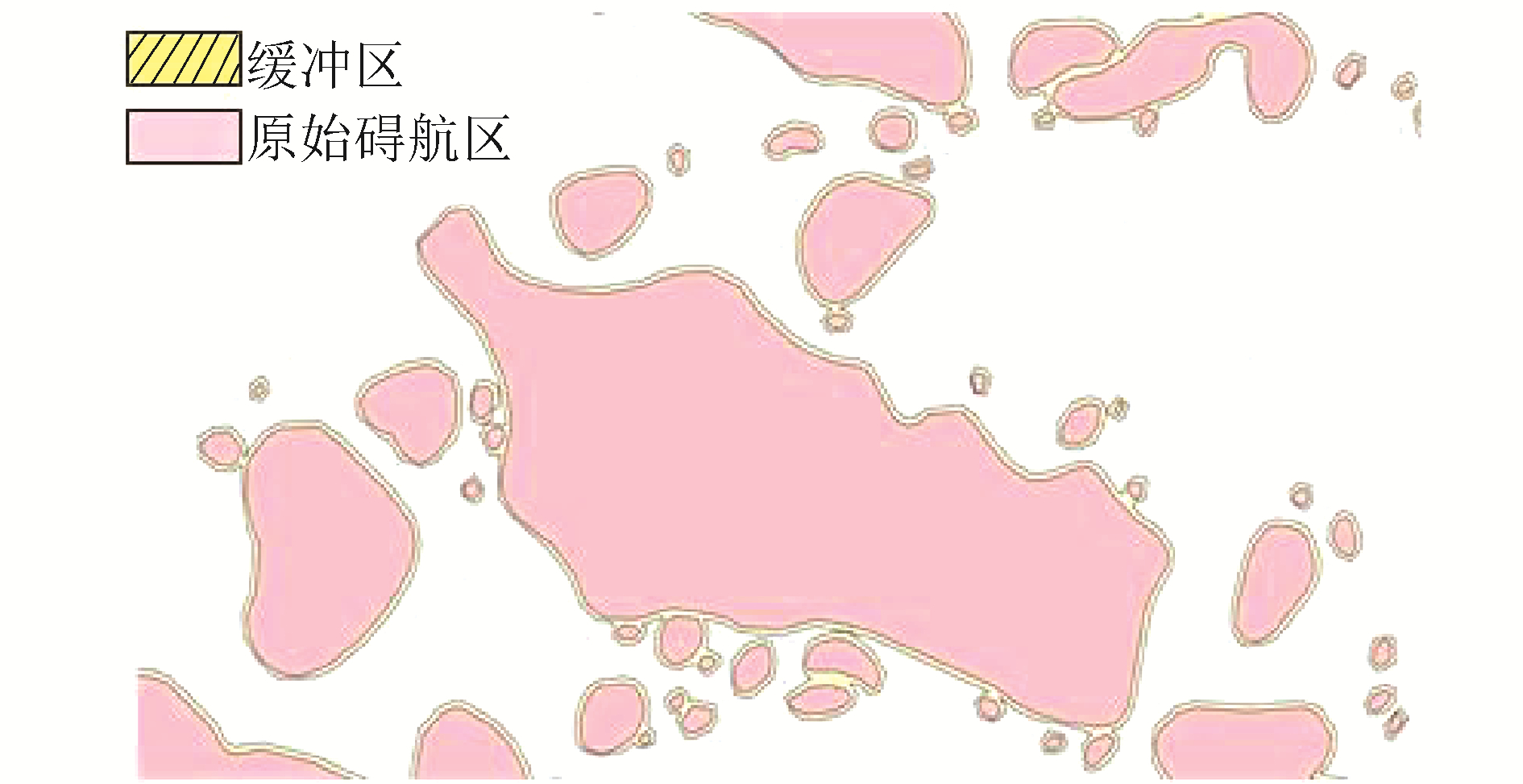Download: 图 1 某海域碍航区表达 Fig. 1 The expression of obstacle areas in one area
1.1.3 基于航路二叉树的航线自动生成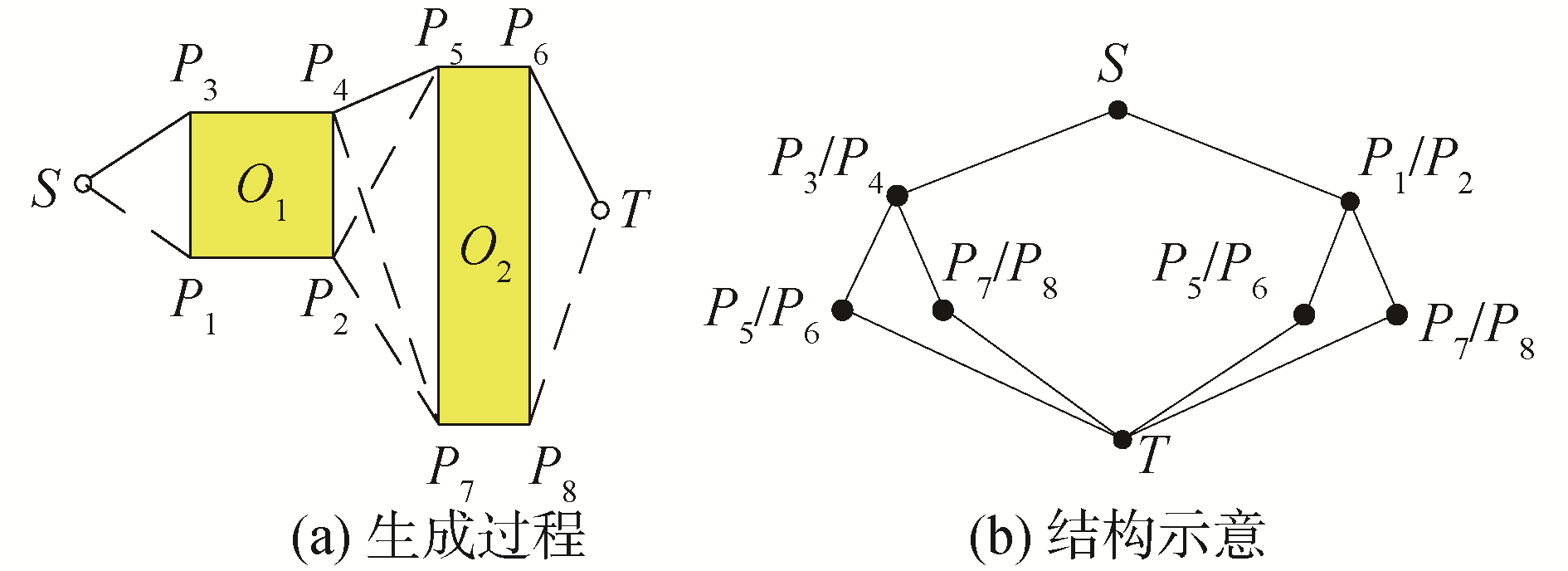Download: 图 2 航路二叉树生成过程及二叉树结构示意 Fig. 2 The process of route binary tree generation and structure of route binary tree
1.2 改进方法的基本思想

1) 多幅海图碍航区数据融合。通过对比同区域不同精度的单幅海图数据，构建多幅海图碍航区数据融合模型，对不同海图的碍航区进行融合；

2) 碍航区数据库建立及增量更新。考虑海图小改正及更新等因素的影响，建立碍航区空间数据库并设计增量更新机制；

3) 效率优化。考虑融合后碍航区数据量激增对算法效率的影响，通过构建航路窗口和改进R树，对碍航区数据进行快速提取、查询，提高航路二叉树算法的运行效率。

1.3 多幅海图碍航区数据融合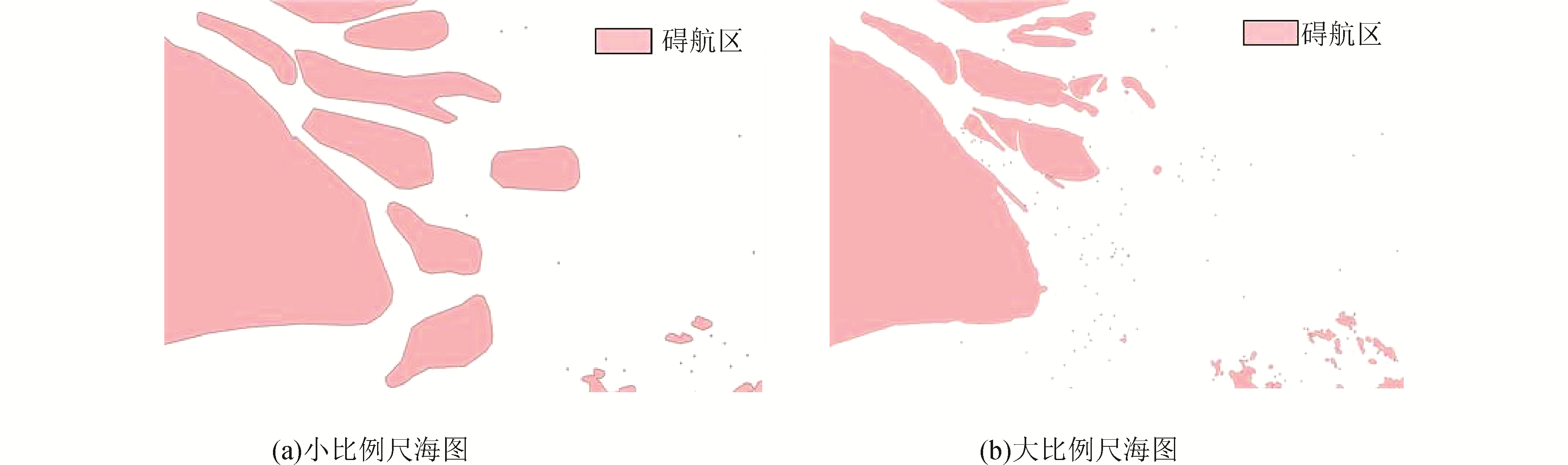Download: 图 3 不同海图在同一海域内的碍航区表达结果 Fig. 3 The expression of different chart′s obstacle areas in the same area

1) 为定量分析海图的精度，定义海图精度指数μ，对海图的精细化程度进行评估。海图精度指数μ在数值上同海图比例尺呈正相关关系。比例尺越大，精度越高：

 $\left\{ {\begin{array}{*{20}{l}} {{S_{({\rm{A}})}} = {F_{\left( {{\varepsilon _1}, {\mu _1}} \right)}} = \left\{ {{a_0}, {a_1}, {a_2}, \cdots , {a_m}} \right\}}\\ {{S_{({\rm{B}})}} = {F_{\left( {{\varepsilon _2}, {\mu _2}} \right)}} = \left\{ {{b_0}, {b_1}, {b_2}, \cdots , {b_n}} \right\}} \end{array}} \right.$ (1)

2) 海图图幅相交区域是指在2幅或多幅海图区域内，有2幅及以上海图同时包含某海域，形成的海域重叠，需要对其进行提取以便下一步进行融合：

 $\left\{ \begin{array}{l} {\varepsilon _0} = {\varepsilon _1} \cap {\varepsilon _2}\\ {S_{\left( {{{\rm{A}}_0}} \right)}} = {F_{\left( {{\varepsilon _0}, {\mu _1}} \right)}} = {S_{({\rm{A}})}} \cap {\varepsilon _0} = \left\{ {{a_i}, {a_{i + 1}} \cdots {a_{i + k}}} \right\}\\ {S_{\left( {{{\rm{B}}_0}} \right)}} = {F_{\left( {{\varepsilon _0}, {\mu _2}} \right)}} = {S_{({\rm{B}})}} \cap {\varepsilon _0} = \left\{ {{a_j}, {a_{j + 1}}, \cdots {a_{j + h}}} \right\} \end{array} \right.$ (2)

3) 获取到交叠海域后，计算非交叠海域的碍航区集合，在航线生成过程中，这部分海域只有某一精度的碍航物数据，不能将其随意舍去：

 $\left\{ \begin{array}{l} {S_{\left( {{{\rm{A}}^\prime }} \right)}} = {F_{\left( {{\varepsilon _1}, {\mu _1}, {t_1}} \right)}} - {F_{\left( {{\varepsilon _0}, {\mu _1}, {t_1}} \right)}} = \\ \;\;\;\;\;\;\;\;\left\{ {{a_0}, {a_1} \cdots {a_{i - 1}}, {a_{i + k + 1}} \cdots {a_m}} \right\}\\ {S_{\left( {{{\rm{B}}^\prime }} \right)}} = {F_{\left( {{\varepsilon _2}, {\mu _2}, {t_2}} \right)}} - {F_{\left( {{\varepsilon _0}, {\mu _2}, {t_2}} \right)}} = \\ \;\;\;\;\;\;\;\;\;\left\{ {{b_0}, {b_1} \cdots {b_{j - 1}}, {b_{j + h + 1}} \cdots {b_n}} \right\} \end{array} \right.$ (3)

4) 依精度对交叠海域内的碍航区集合进行替换，值得注意的是，2幅或多幅海图之间的交叠区域可能不存在，这时需要将其都纳入碍航物集合范围内：

 ${S_{({\rm{C}})}} = \left\{ \begin{array}{l} {S_{\left( {{{\rm{A}}^\prime }} \right)}} + {S_{\left( {{{\rm{B}}^\prime }} \right)}} + {S_{\left( {{{\rm{A}}_0}} \right)}} = {F_{\left( {{\varepsilon _1}, {\mu _1}} \right)}} + {F_{\left( {{\varepsilon _2}, {\mu _2}} \right)}} - \\ \;\;\;\;\;\;\;\;\;\;\;{F_{\left( {{\varepsilon _0}, {\mu _2}} \right)}}, {\varepsilon _0} \ne 0, {\mu _1} \ge {\mu _2}\\ {S_{\left( {{{\rm{A}}^\prime }} \right)}} + {S_{\left( {{{\rm{B}}^\prime }} \right)}} + {S_{\left( {{{\rm{B}}_0}} \right)}} = {F_{\left( {{\varepsilon _1}, {\mu _1}} \right)}} - {F_{\left( {{s_0}, {\mu _1}} \right)}} + \\ {F_{\left( {{s_2}, {\mu _2}} \right)}}, {\varepsilon _0} \ne 0, {\mu _2} > {\mu _1}\\ {S_{\left( {{{\rm{A}}^\prime }} \right)}} + {S_{\left( {{{\rm{B}}^\prime }} \right)}} = {F_{\left( {{\varepsilon _1}, {\mu _1}} \right)}} + {F_{\left( {{\varepsilon _2}, {\mu _2}} \right)}}, {\varepsilon _0} = 0 \end{array} \right.$ (4)

1.4 碍航区数据库建立及增量更新

 $S = F(\varepsilon , \mu , t)$ (5)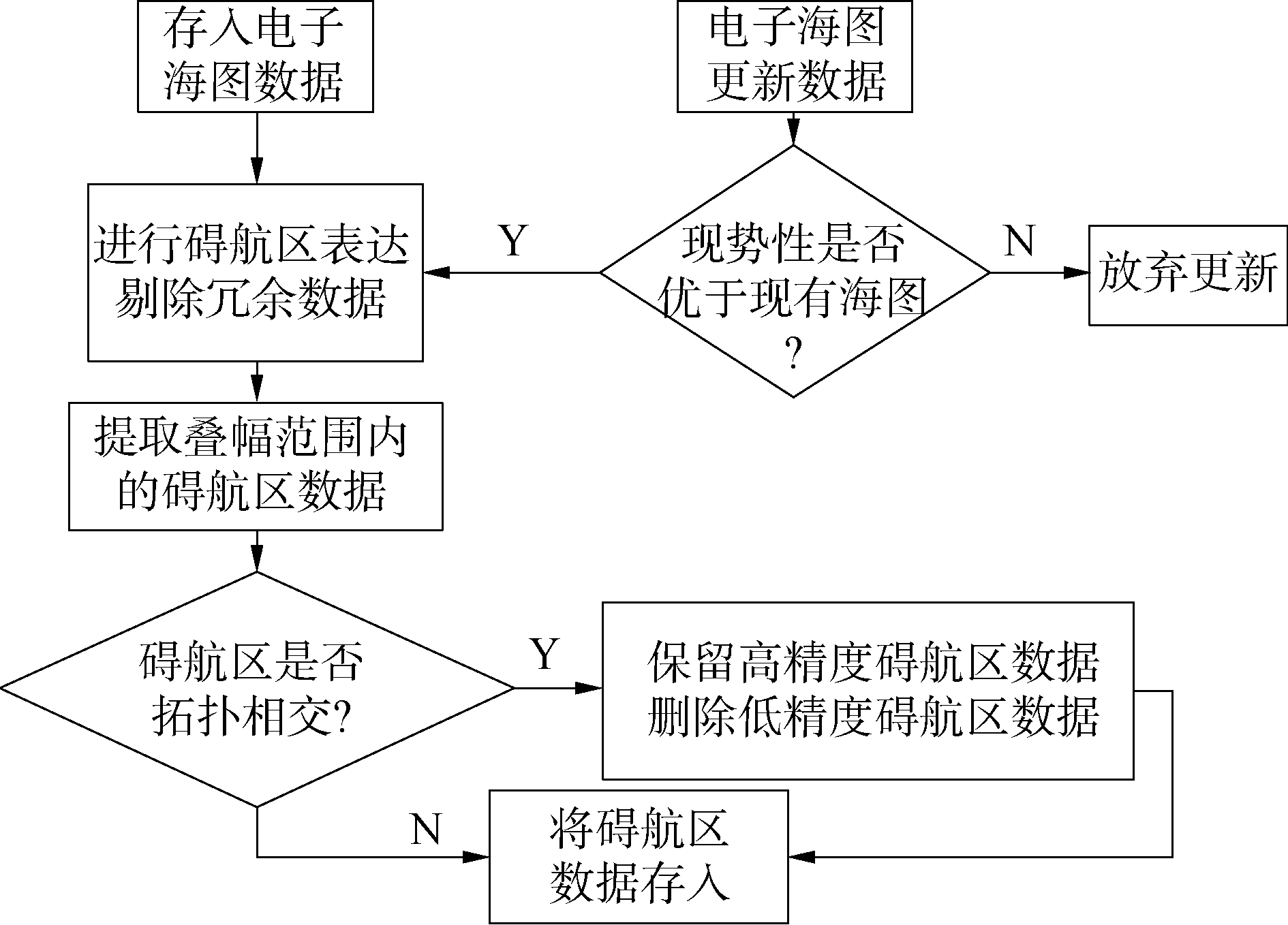Download: 图 4 碍航区数据融合更新流程 Fig. 4 The flow-diagram of obstacle data fusion and update

1) 在现有海图数据的基础上，将海图数据存入空间数据库中。

2) 按图幅对空间数据库中的海图进行读取，依据1.1.2节中所述方式对其进行单幅海图的碍航区表达，同时剔除数据库中与航行无关的要素以减少数据冗余。

3) 依据1.3节中所述方式进行多幅海图的碍航区融合，获取海图图幅范围，提取叠幅海域的碍航区数据，依精度对其进行替换，保留高精度数据，将低精度数据从数据库中删除。形成顾及精度和范围的海图数据。

4) 若存在新的海图数据，则定量分析其版本号、出版时间等现势性要素，判断其是否优于现有海图数据。对更新海图完成表达后重复步骤2)、3)，对空间数据库中的碍航区数据进行更新，保证数据的现势性。

1.5 航路二叉树算法的效率优化

1.5.1 面向航路窗口的碍航区快速提取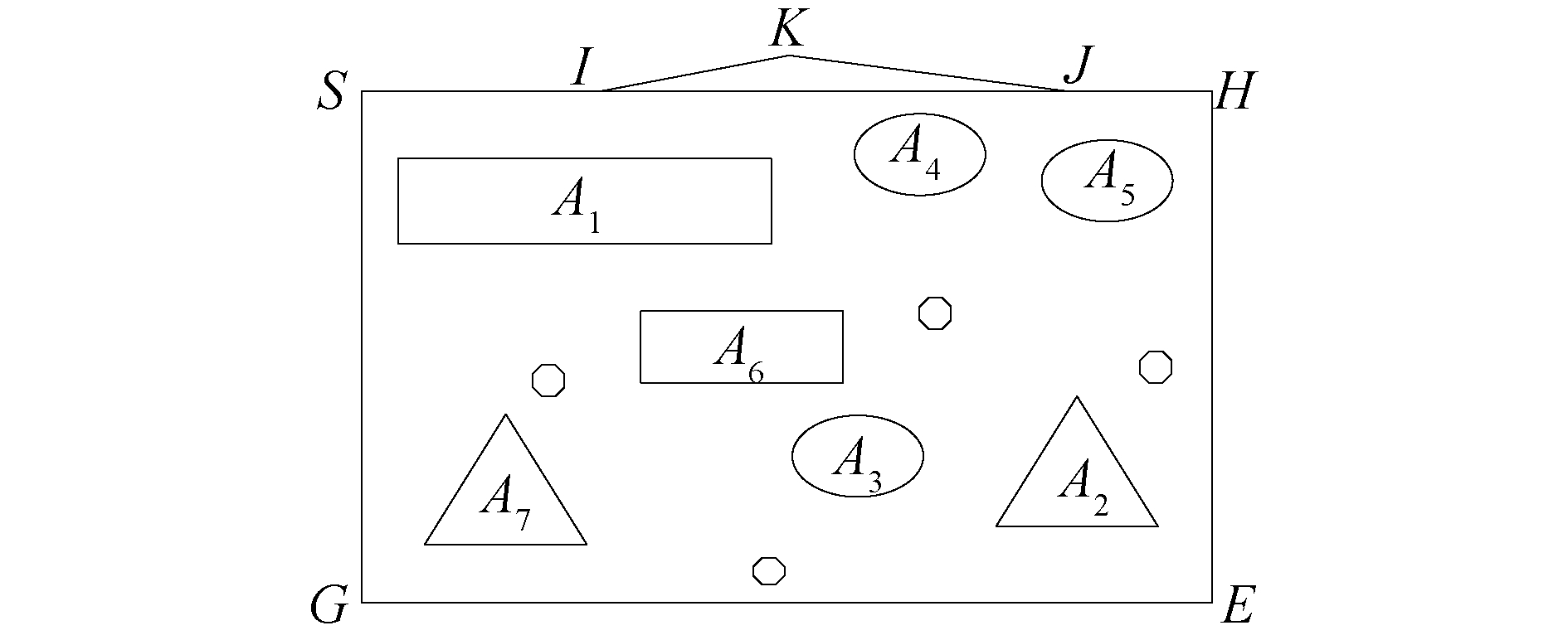Download: 图 5 航路窗口及其所含碍航区 Fig. 5 Route window and its obstacle areas
1.5.2 基于改进R树索引的碍航区快速查询

R树索引是目前应用非常广泛的一种空间数据索引，实践证明相对于其他空间索引，R树索引对于大量空间数据有更加出色的表现。常规的R树索引由根节点、中间节点和叶节点组成，中间节点代表空间数据中的一个矩形，该矩形是其所有叶节点的最小外接矩形(MBR)，并不是实际世界中的地理对象，叶节点则是具有现实意义的地理实体。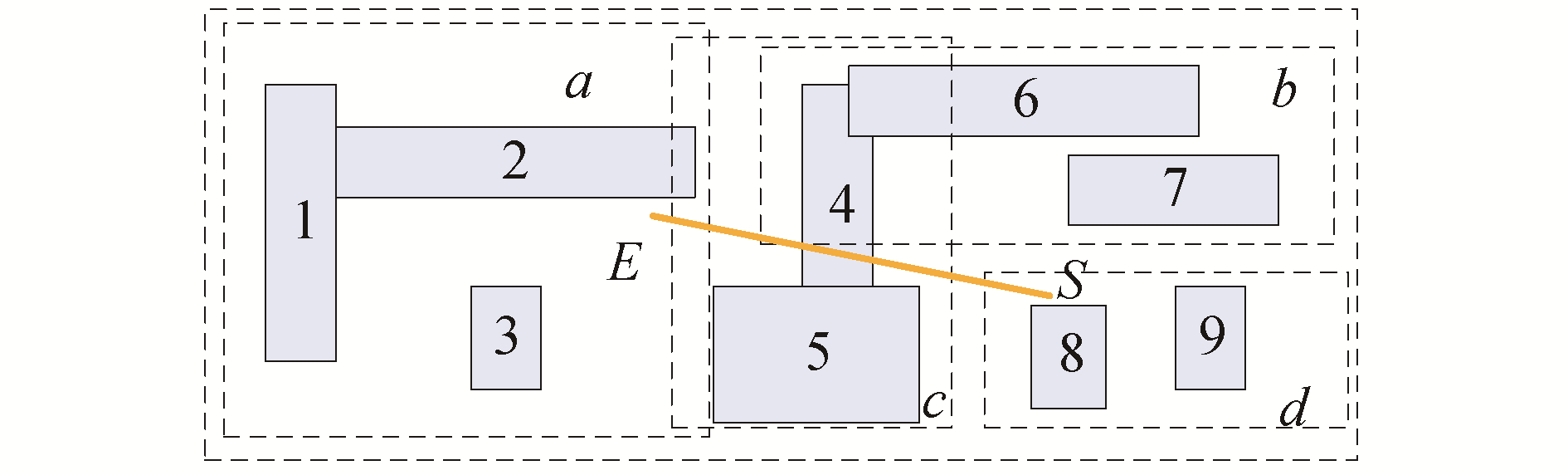Download: 图 6 传统R树中的空间查询示意 Fig. 6 The diagram of spatial query in traditional R-tree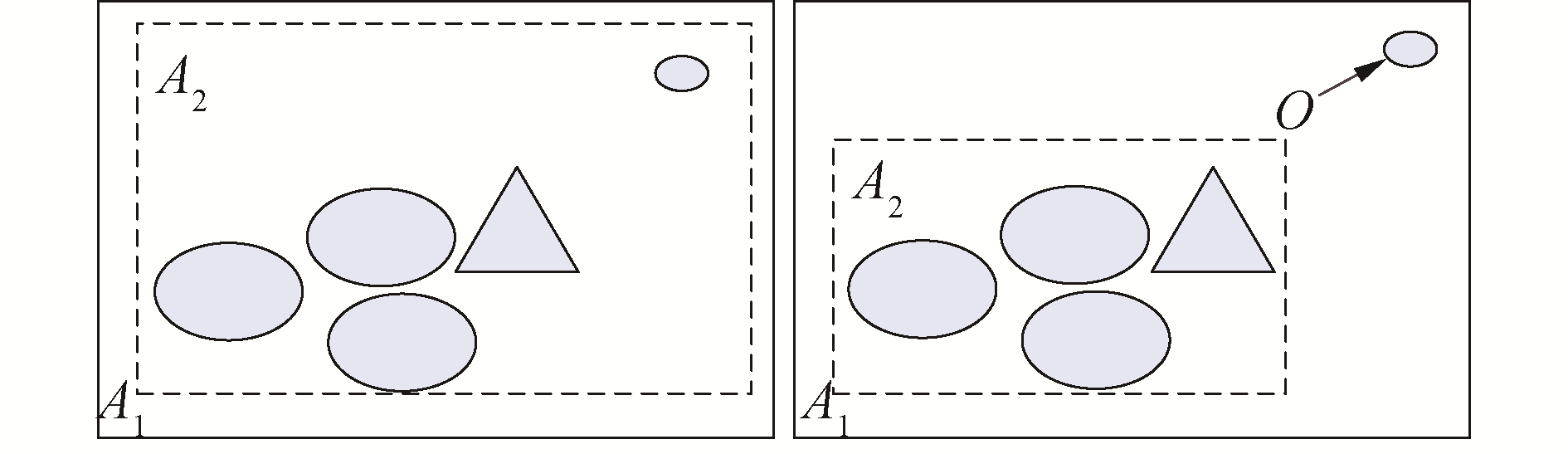Download: 图 7 节点分裂模型 Fig. 7 Node splitting model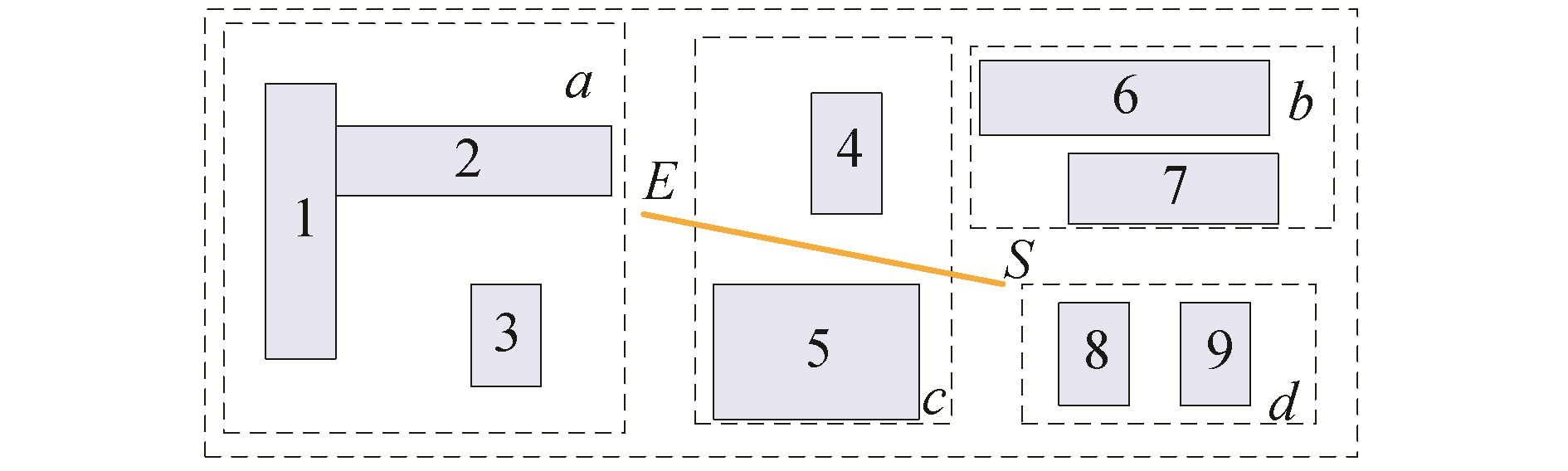Download: 图 8 改进后的R树空间查询示意 Fig. 8 The diagram of space query in improved R tree
2 实验与分析 2.1 碍航区生成质量比对分析表 1 碍航区测试电子海图 Table 1 Electronic charts used to make obstacle areas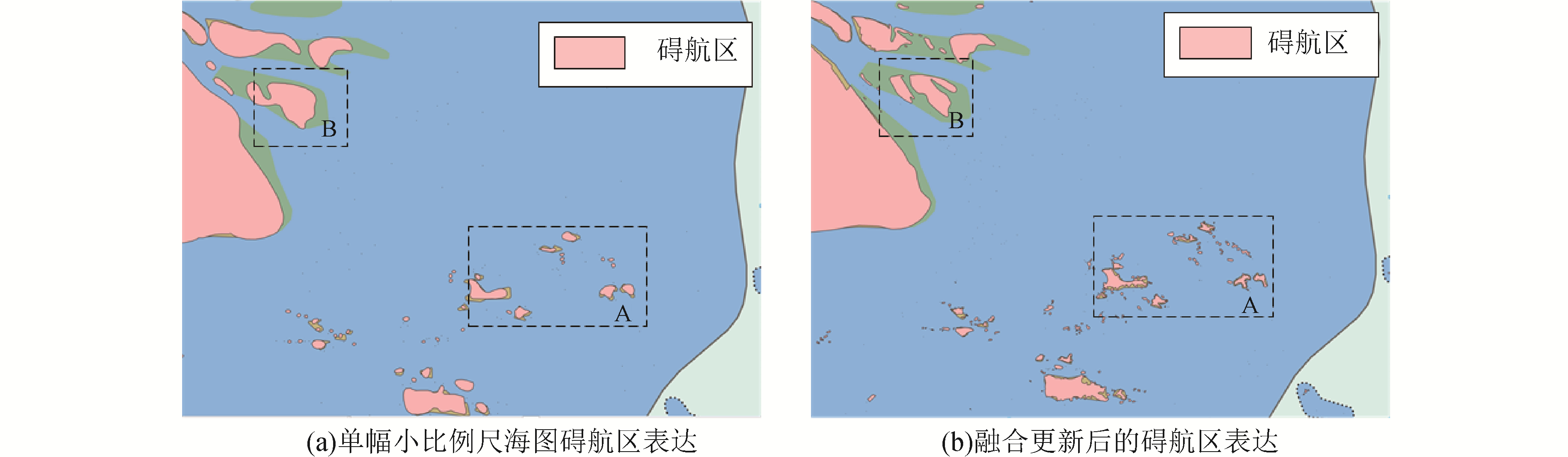Download: 图 9 碍航区生成结果 Fig. 9 Result of obstacle areas expression

2.2 航线生成质量比对分析表 2 航线生成测试电子海图 Table 2 Electronic charts used for route makingDownload: 图 10 航线自动生成结果 Fig. 10 Results of route making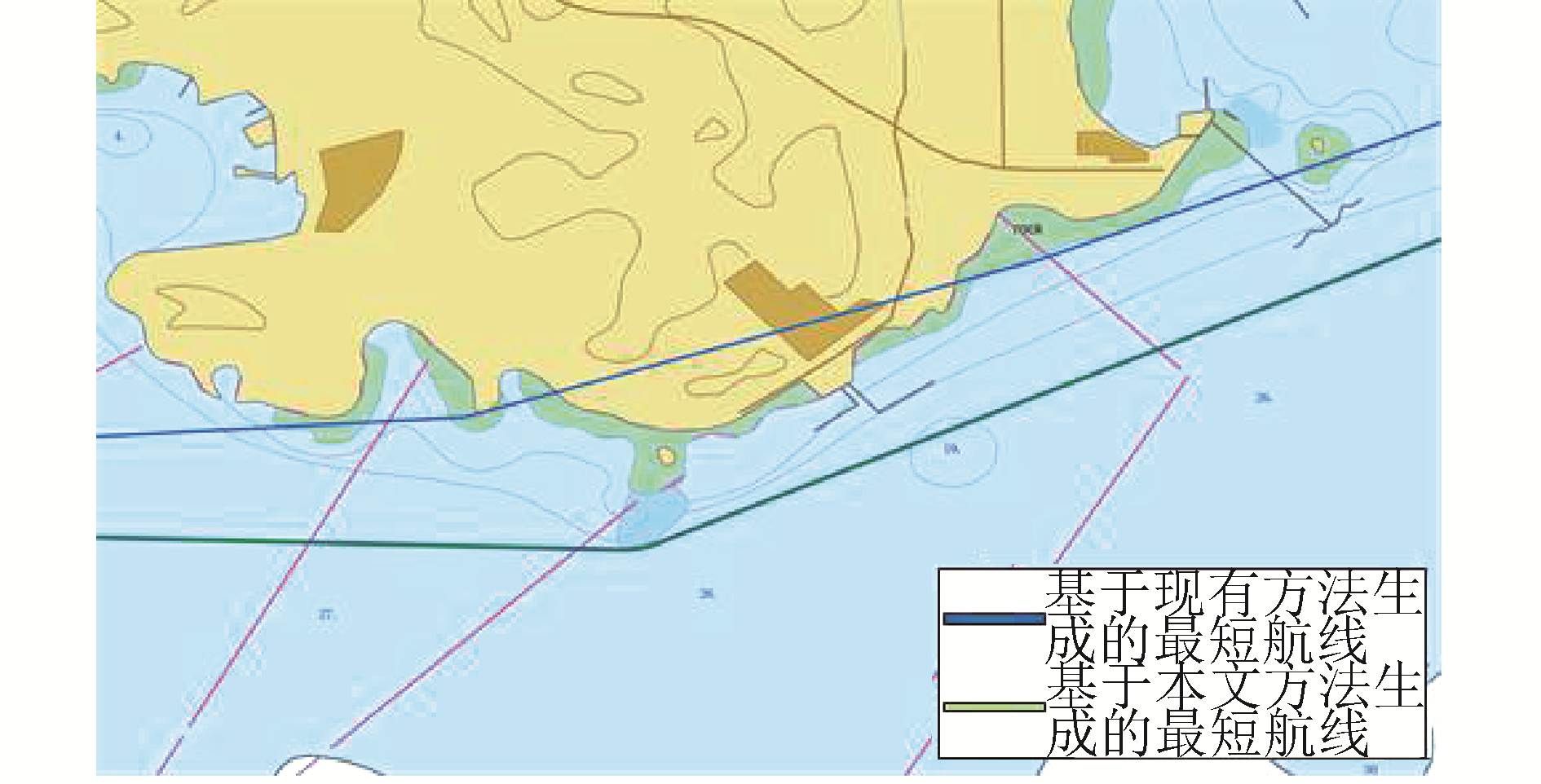Download: 图 11 某海域航线对比 Fig. 11 The comparison of routes in one area表 3 不同方法可行性及可靠性比对 Table 3 Feasibility and reliability comparison of different methods
2.3 航线生成效率比对分析表 4 不同方法算法效率情况 Table 4 The comparison of different methods in efficiency

3 结论

1) 与现有基于单幅海图的碍航区表达方式相比，本文所提通过分析不同海图的精度、现势性差异，对多幅海图的碍航区进行数据融合、所构建的碍航区对真实地理环境表达的准确程度高。

2) 与现有航路二叉树方法的应用局限于单幅海图相比，本文所提方法能够实现跨海图图幅的航线自动生成论在航程上还是可靠程度上，都得到长足提升。在航程和可靠性上都有了明显的提高。

3) 同现有航路自动生成方法相比，在大量碍航区数据下，本文所提航路窗口、改进R树方式对航路自动生成效率进行了极大改进，航线生成时间大大缩短。

  张立华, 朱庆, 刘雁春, 等. 电子海图平台下的航线自动设计方法[J]. 大连海事大学学报, 2007, 33(3): 109-112. ZHANG Lihua, ZHU Qing, LIU Yanchun, et al. A method for automatic routing based on ECDIS[J]. Journal of Dalian Maritime University, 2007, 33(3): 109-112. DOI:10.3969/j.issn.1006-7736.2007.03.024 (0)  曹鸿博, 张立华, 贾帅东, 等. 电子海图最短距离航线自动生成的改进方法[J]. 武汉大学学报(信息科学版), 2011, 36(9): 1107-1110. CAO Hongbo, ZHANG Lihua, JIA Shuaidong, et al. An Improved method for automatically building shortest route based on electronic chart[J]. Geomatics and Information Science of Wuhan University, 2011, 36(9): 1107-1110. (0)  CHANG K Y, JAN G E, PARBERRY I. A method for searching optimal routes with collision avoidance on raster charts[J]. The journal of navigation, 2004, 56(3): 371-384. (0)  王德春, 陈利敏, 张孝芳. 基于A*算法的舰船最佳航线选择[J]. 青岛大学学报(自然科学版), 2005, 18(4): 10-13. WANG Dechun, CHEN Limin, ZHANG Xiaofang. Selecting ship's optimum route using A* algorithm[J]. Journal of Qingdao University (natural science edition), 2005, 18(4): 10-13. DOI:10.3969/j.issn.1006-1037.2005.04.003 (0)  叶清, 郁振伟. 改进最短路径算法在最佳航线选择中的应用[J]. 中国航海, 2003(2): 15-17. YE Qing, YU Zhengwei. The improved shortcut algorithm and it's application in selecting ship's optimum route[J]. Navigation of China, 2003(2): 15-17. DOI:10.3969/j.issn.1000-4653.2003.02.005 (0)  王科.基于电子海图的航线设计研究[D].大连: 海军大连舰艇学院, 2004. WANG Ke. A study for designing navigation route based on ECDIS[D]. Dalian: Dalian naval Academic, 2004. (0)  ANEL A M. Network shortest path application for optimum track ship routing[D]. California: Naval Postgraduate School, 2005. (0)  李源惠, 潘明阳, 吴娴. 基于动态网格模型的航线自动生成算法[J]. 交通运输工程学报, 2007, 7(3): 34-39. LI Yuanhui, PAN Mingyang, WU Xian. Automatic creating algorithm of route based on dynamic grid model[J]. Journal of traffic and transportation engineering, 2007, 7(3): 34-39. DOI:10.3321/j.issn:1671-1637.2007.03.008 (0)  SZLAPCZYNSKI R. A new method of ship routing on raster grids, with turn penalties and collision avoidance[J]. The journal of navigation, 2006, 59(1): 27-42. (0)  汪柱, 李树军, 张立华, 等. 基于航路二叉树的航线自动生成方法[J]. 武汉大学学报(信息科学版), 2010, 35(4): 407-410. WANG Zhu, LI Shujun, ZHANG Lihua, et al. A method for automatic routing based on route binary tree[J]. Geomatics and Information Science of Wuhan University, 2010, 35(4): 407-410. (0)  王涛, 张立华, 彭认灿, 等. 顾及航道宽度的最短距离航线自动生成方法[J]. 海洋测绘, 2016, 36(3): 29-31, 36. WANG Tao, ZHANG Lihua, PENG Rencan, et al. A method for automatically generating the shortest distance route based on electronic navigational chart considering channel width[J]. Hydrographic surveying and charting, 2016, 36(3): 29-31, 36. DOI:10.3969/j.issn.1671-3044.2016.03.007 (0)  王涛, 张立华, 彭认灿, 等. 考虑转向限制的电子海图最短距离航线自动生成方法[J]. 哈尔滨工程大学学报, 2016, 37(7): 923-929. WANG Tao, ZHANG Lihua, PENG Rencan, et al. Automatic generation of the shortest route of an electronic navigational chart considering turning restrictions[J]. Journal of Harbin Engineering University, 2016, 37(7): 923-929. (0)  张莉, 金一丞, 汪柱, 等. 电子海图的航线多尺度生成方法[J]. 测绘科学, 2011, 36(5): 197-199. ZHANG Li, JIN Yichen, WANG Zhu, et al. A method for multi-scale routing based on ECDIS[J]. Science of surveying and mapping, 2011, 36(5): 197-199. (0)  张莉, 王义涛. 一种基于海图水深的碍航区自动提取方法[J]. 海洋技术, 2010, 29(3): 69-72. ZHANG Li, WANG Yitao. A method for automatic acquisition of obstacle areas based on the depth in a chart[J]. Ocean technology, 2010, 29(3): 69-72. DOI:10.3969/j.issn.1003-2029.2010.03.016 (0)  张浩, 栾俊, 纪宏宇, 等. 一种碍航区自动提取的方法[J]. 海洋测绘, 2015, 35(6): 55-57. ZHANG Hao, LUAN Jun, JI Hongyu, et al. A method for constructing obstacle areas[J]. Hydrographic surveying and charting, 2015, 35(6): 55-57. DOI:10.3969/j.issn.1671-3044.2015.06.014 (0)  张立华, 朱庆, 张安民, 等. 一种智能的最短航线构建方法[J]. 测绘学报, 2008, 37(1): 114-120. ZHANG Lihua, ZHU Qing, ZHANG Anmin, et al. An intelligent method for the shortest routing[J]. Acta geodaetica et cartographica sinica, 2008, 37(1): 114-120. DOI:10.3321/j.issn:1001-1595.2008.01.020 (0)  贾帅东, 张立华, 彭认灿, 等. 确保水深模型航海安全性的深度保证率控制方法[J]. 交通运输工程学报, 2015, 15(5): 101-109. JIA Shuaidong, ZHANG Lihua, PENG Rencan, et al. Control method of probability of adequate depth ensuring navigation safety of depth model[J]. Journal of traffic and transportation engineering, 2015, 15(5): 101-109. DOI:10.3969/j.issn.1671-1637.2015.05.013 (0)  陈惠荣.海图设计自动化关键技术研究[D].大连海事大学, 2011. CHEN Huirong. Research on key techniques of chart design automation[D]. Dalian: Dalian Maritime University, 2011. http://cdmd.cnki.com.cn/Article/CDMD-10151-1012261155.htm (0)  汤青慧.基于电子海图的航线规划方法研究[D].中国海洋大学, 2011. TANG Qinghui. Research on route planning method based on electronic chart[D]. Qingdao: Ocean University of China, 2011. http://cdmd.cnki.com.cn/Article/CDMD-10423-1011231344.htm (0)  邓红艳, 武芳, 翟仁健, 等. 一种用于空间数据多尺度表达的R树索引结构[J]. 计算机学报, 2009, 32(1): 177-184. DENG Hongyan, WU Fang, ZHAI Renjian, et al. R-tree index structure for multi-scale representation of spatial data[J]. Chinese journal of computers, 2009, 32(1): 177-184. (0)